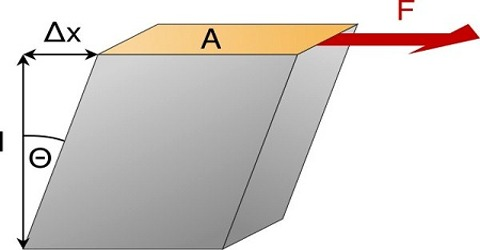# Rigidity Modulus

Rigidity modulus

Within the elastic limit, the ratio of the shearing stress and shearing strain is constant. This constant is called rigidity modulus. It is expressed as ‘n’

Rigidity modulus, n = [shearing stress / shearing strain]

Explanation: If a force F applied tangentially on the surface of the body the shearing strain of the surface is θ, an area of the surface is A, then,

n = [(F/A); or, n = F / Aθ.

Unit and dimension of rigidity modulus are identical to those of Young’s modulus. The modulus of rigidity is denoted by G and expressed in pascals. Modulus of rigidity value of a material is determined by a torsion test.Modulus of Rigidity can be determined by performing a tensile stress test where stress vs strain is plotted. The slope of the line is equal to the Modulus of Rigidity. Rigidity modulus of iron is 7.7 x 1010 Nm-2 means that in order to produce one-radian angular rotation the upper surface of the cube of iron the force needed to apply on the upper surface of the cube is: 7.7 x 1010 N.